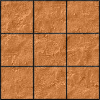#### You may also like### Pyramids

What are the missing numbers in the pyramids?### Paving the Way

A man paved a square courtyard and then decided that it was too small. He took up the tiles, bought 100 more and used them to pave another square courtyard. How many tiles did he use altogether?### Chess

What would be the smallest number of moves needed to move a Knight from a chess set from one corner to the opposite corner of a 99 by 99 square board?

# Sum and Differences

##### Age 11 to 14 Short Challenge Level:

Answer: $28, 30, 42$

Using algebra
Middle number is $n$

\begin{align}n-2\ \ +\ \ n\ \ +\ \ n+12 &= 100\\ \Rightarrow 3n+10&=100\\ \Rightarrow 3n&=90\\ \Rightarrow n&=30\end{align}

So $n=30$, $n+12=42$ and $n-2=28$.

Guess and check - differences
Try some numbers which are the right distances apart

\begin{align}30 + 32 + 44 &= 106\\ &=100 + 6\\ &= 100+3\times2\end{align}
$\therefore (30-2)+(32-2)+(44-2)$ should work

$28+30+42=100$

Guess and check - sum
Try some numbers which add up to $100$

$33+33+34=100$

Need to make largest number larger, always balancing out changes:
$(33-2)+\ \quad33\ \quad+(34+2)=31+33+36=100$

$(31-1)+(33-1)+(36+2)=30+32+38=100$

$(30-2)+(32-2)+(38+4)=28+30+42=100$

You can find more short problems, arranged by curriculum topic, in our short problems collection.